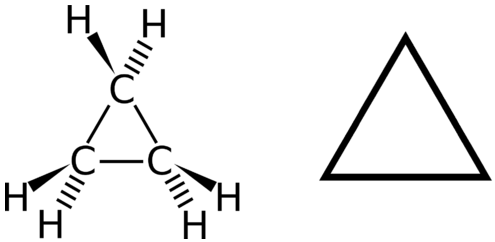# 12.9: Cycloalkanes

$$\newcommand{\vecs}{\overset { \rightharpoonup} {\mathbf{#1}} }$$ $$\newcommand{\vecd}{\overset{-\!-\!\rightharpoonup}{\vphantom{a}\smash {#1}}}$$$$\newcommand{\id}{\mathrm{id}}$$ $$\newcommand{\Span}{\mathrm{span}}$$ $$\newcommand{\kernel}{\mathrm{null}\,}$$ $$\newcommand{\range}{\mathrm{range}\,}$$ $$\newcommand{\RealPart}{\mathrm{Re}}$$ $$\newcommand{\ImaginaryPart}{\mathrm{Im}}$$ $$\newcommand{\Argument}{\mathrm{Arg}}$$ $$\newcommand{\norm}{\| #1 \|}$$ $$\newcommand{\inner}{\langle #1, #2 \rangle}$$ $$\newcommand{\Span}{\mathrm{span}}$$ $$\newcommand{\id}{\mathrm{id}}$$ $$\newcommand{\Span}{\mathrm{span}}$$ $$\newcommand{\kernel}{\mathrm{null}\,}$$ $$\newcommand{\range}{\mathrm{range}\,}$$ $$\newcommand{\RealPart}{\mathrm{Re}}$$ $$\newcommand{\ImaginaryPart}{\mathrm{Im}}$$ $$\newcommand{\Argument}{\mathrm{Arg}}$$ $$\newcommand{\norm}{\| #1 \|}$$ $$\newcommand{\inner}{\langle #1, #2 \rangle}$$ $$\newcommand{\Span}{\mathrm{span}}$$

## Learning Objectives

• Identify the structures of cycloalkanes.

A cyclic hydrocarbon is a hydrocarbon in which the carbon chain joins to itself in a ring. A cycloalkane is a cyclic hydrocarbon in which all of the carbon-carbon bonds are single bonds and each carbon is bonded to two hydrogen atoms, they are saturated compounds. Cycloalkanes have the general formula $$\ce{C_{n}H_{2n}}$$. The simplest of these cyclic hydrocarbons, cyclopropane, has the formula C3H6, which makes a three-carbon ring.Figure $$\PageIndex{1}$$: Cyclopropane is the simplest cycloalkane. Its highly strained geometry makes it rather unstable and highly reactive.

The structural formulas of cyclic hydrocarbons can be represented in multiple ways, two of which are shown above. Each atom can be shown as in the structure on the left from the figure above. A convenient shorthand is to omit the element symbols and only show the shape, as in the triangle on the right. Carbon atoms are understood to be the vertices of the triangle.

The carbon atoms in cycloalkanes have a bond angle of $$109.5^\text{o}$$. However, an examination of the cyclopropane structure shows that the triangular structure results in a $$\ce{C-C-C}$$ bond angle of $$60^\text{o}$$. This deviation from the ideal angle is called ring strain and makes cyclopropane a fairly unstable and reactive molecule. Ring strain is decreased for cyclobutane, with a bond angle of $$90^\text{o}$$, but is still significant. Cyclopentane has a bond angle of about $$108^\text{o}$$. This minimal ring strain for cyclopentane makes it a more stable compound.Cyclohexane is a six-carbon cycloalkane, shown below.All three of the depictions of cyclohexane above are somewhat misleading, because the molecule is not planar. In order to reduce the ring strain and attain a bond angle of approximately $$109.5^\text{o}$$, the molecule is actually puckered.

The ring structure in cycloalkanes also prevents rotation around the carbon–carbon bonds without breaking open the ring, thus they are more rigid and less flexible than acyclic alkanes. This property is called restricted rotation.

## Note To Your Health: Cyclopropane as an Anesthetic

With its boiling point of −33°C, cyclopropane is a gas at room temperature. It is also a potent, quick-acting anesthetic with few undesirable side effects in the body. It is no longer used in surgery, however, because it forms explosive mixtures with air at nearly all concentrations.

12.9: Cycloalkanes is shared under a CC BY-NC-SA 3.0 license and was authored, remixed, and/or curated by LibreTexts.